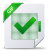# Relaxation of two-phase flows via the Born-Infeld system

Relaxation of two-phase flows via the Born-Infeld system

The aim of this paper is to call the reader’s attention to an original scheme for solving special scalar conservation laws and drift-°ux two-phase °ow models. The scalar conservation law is ubut unlike Xin and Jin’s approach , it is more \natural » insofar as there is no free parameter involved. Instead, it makes use of the 2 £ 2 Born-Infeld system [12, 59] and enables one to achieveInitially, we were interested in relaxation methods for solving a two-phase °ow model. We devised an explicit scheme  and its semi-implicit version . When we tried to extend these schemes to the multi-component case, a major di±culty arised, the essence of which boils down to the following prototype.are the left and right state of a local Riemann problem.Xin and Jin’s method is what we refer to as the \traditional » or \brute-force » relaxation strategy. Unfortunately, when extended to our initial multi-component system, the traditional relaxation is spurred with a division-by-zero problem for the computation of the variables W can be interpreted as a relaxation system for (D.1). Then, we elaborate on various properties of this unexpected and non-standard system. In particular, the Riemann problem associated with it is readily solved, which provides us with bounded values for w. This feature is a tremendous advantage of (D.10) over (D.6). It is important to notice that the characteristic ¯elds of the system D.10 are linearly degenerate, which is a central property, shared with (D.6). This advantage is one of a series of other enjoyable properties that will be reviewed afterward.

This strategy has been applied on the drift-°ux model which is a conservative two-phase °ow model. We will verify that the classical Zuber-Findlay slip law obeys to a Whitham-like stability condition. In pratice, the relaxation scheme behave well on Riemann problems for several slip laws that have a physical meaning which will be highlighted by the numerical experiments. This paper is organized as follows. Sections 2 and 3 are devoted to the scalar conservation laws while sections 4 and 5 are devoted to the drift-°ux model. In section 2, we present the properties of the numerical scheme for the scalar conservation law, including the solution of the Riemann problem associated and the stability properties. Section 3 is devoted to the numerical results for the scalar conservation law. In section 4, we show how to construct a relaxation method on the drift-°ux model and the numerical experiments for this model are shown in section 5. Section 6 concludes.As was already the case for other relaxation schemes [15, 17, 20, 36], linear degeneracy of the eigen¯elds is a crucial property. Among other nice features, it makes Riemann problems easy to solve and guarantees equivalence between weak solutions to various conservative and non-conservative forms of the system.

Lire sur cLicours.com :  Codes d’investissement et concept du regime exorbitant

= max(v; 0) stand for the negative and positive parts of any real number v 2 R.Proof- By virtue of linear degeneracy, shock curves coincide with rarefaction curves. In the (W; Z)- plane, W -curves are vertical lines, while Z-curves are horizontal lines. Riemann problems can thus be solved \by hand, » depending on the positions of the two initial states, as depicted in Fig. D.2 and D.3. There are 4 di®erent cases, which can be divided into 2 major cases (1-2), each having 2 subcases (a-b). For each major case, a synthetic formula can be found to encapsulate the 2 subcases. ¤To prove that (D.42) implies (D.41), we proceed in a similar way. Now, if we have only weak solutions, equivalence between (D.42) and (D.41) is still valid because of the linear degeneracy of the eigen¯elds. ¤The conservative form (D.42) is de¯nitely the system actually solved by the scheme (D.32), equipped with the °ux (D.30). The relaxed slip variable G comes in handier than the °ux F . Had we kept working with F instead of G, the writing of the system would have been more cumbersome.Télécharger le document complet